Anzeige

# Dynamical t/U expansion for the doped Hubbard model

5. Mar 2020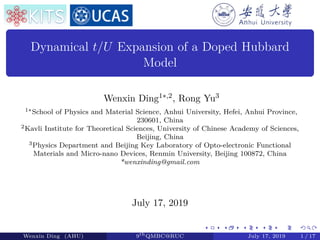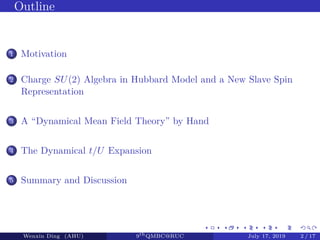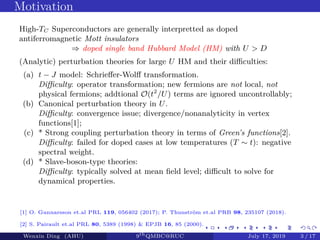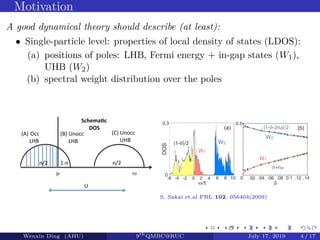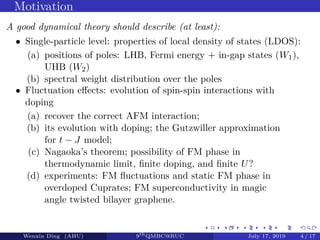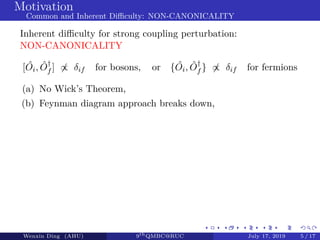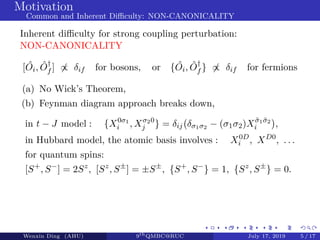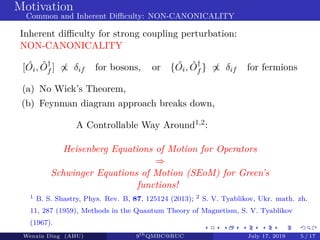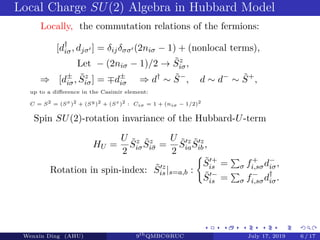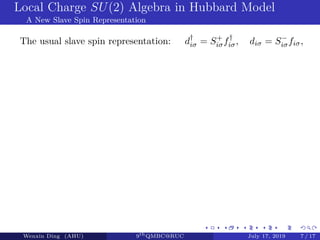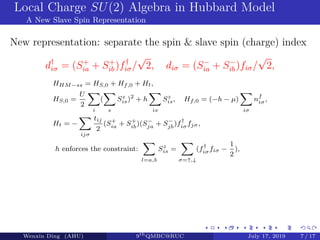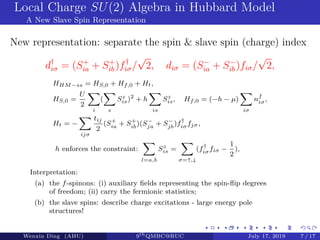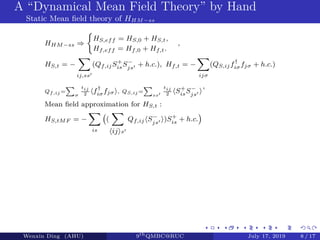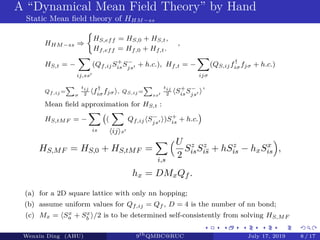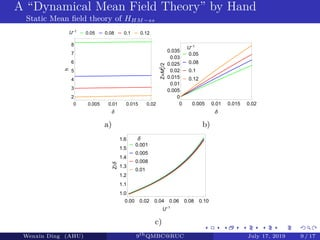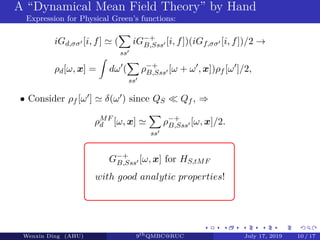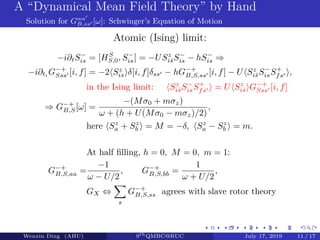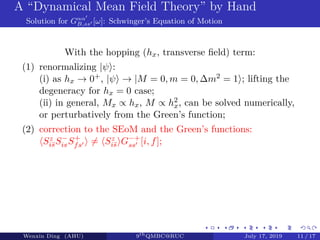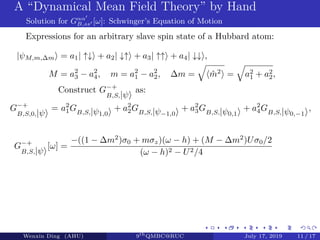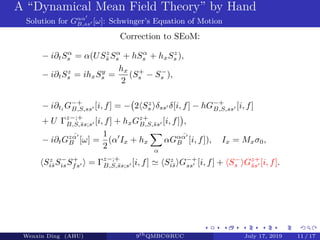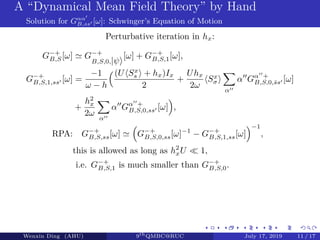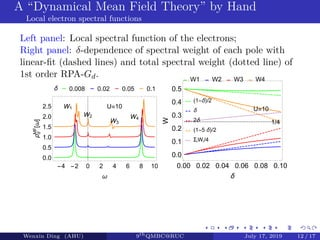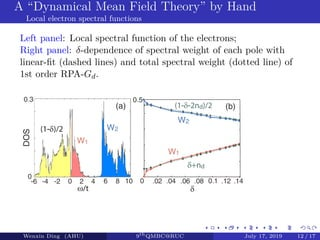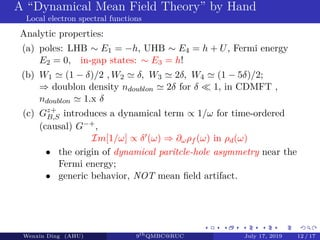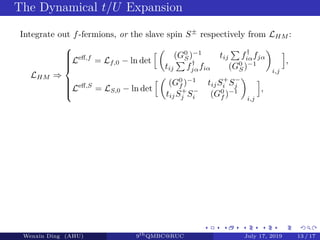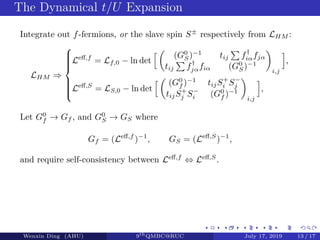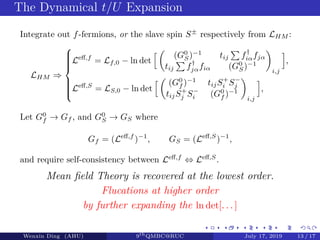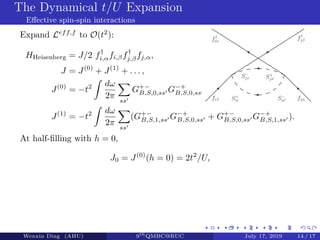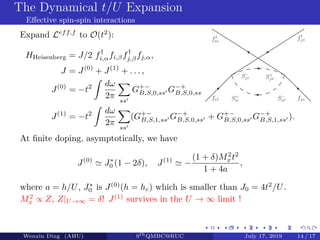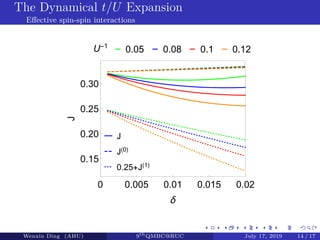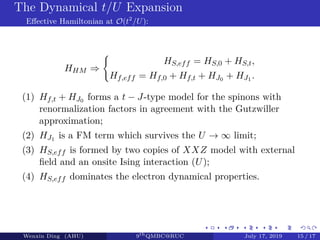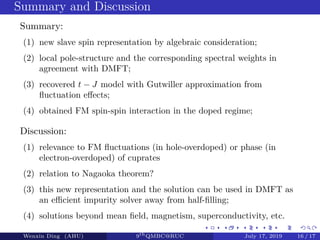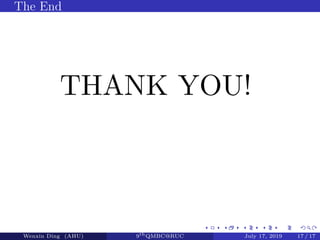1 von 33
Anzeige

### Dynamical t/U expansion for the doped Hubbard model

1. Dynamical t/U Expansion of a Doped Hubbard Model Wenxin Ding1∗,2, Rong Yu3 1∗School of Physics and Material Science, Anhui University, Hefei, Anhui Province, 230601, China 2Kavli Institute for Theoretical Sciences, University of Chinese Academy of Sciences, Beijing, China 3Physics Department and Beijing Key Laboratory of Opto-electronic Functional Materials and Micro-nano Devices, Renmin University, Beijing 100872, China *wenxinding@gmail.com July 17, 2019 Wenxin Ding (AHU) 9th QMBC@RUC July 17, 2019 1 / 17
2. Outline 1 Motivation 2 Charge SU(2) Algebra in Hubbard Model and a New Slave Spin Representation 3 A “Dynamical Mean Field Theory” by Hand 4 The Dynamical t/U Expansion 5 Summary and Discussion Wenxin Ding (AHU) 9th QMBC@RUC July 17, 2019 2 / 17
3. Motivation Wenxin Ding (AHU) 9th QMBC@RUC July 17, 2019 3 / 17 High-TC Superconductors are generally interpretted as doped antiferromagnetic Mott insulators ⇒ doped single band Hubbard Model (HM) with U > D (Analytic) perturbation theories for large U HM and their diﬃculties: (a) t − J model: Schrieﬀer-Wolﬀ transformation. Diﬃculty: operator transformation; new fermions are not local, not physical fermions; addtional O(t2 /U) terms are ignored uncontrollably; (b) Canonical perturbation theory in U. Diﬃculty: convergence issue; divergence/nonanalyticity in vertex functions; (c) * Strong coupling perturbation theory in terms of Green’s functions. Diﬃculty: failed for doped cases at low temperatures (T ∼ t): negative spectral weight. (d) * Slave-boson-type theories: Diﬃculty: typically solved at mean ﬁeld level; diﬃcult to solve for dynamical properties.  O. Gunnarsson et.al PRL 119, 056402 (2017); P. Thunström et.al PRB 98, 235107 (2018).  S. Pairault et.al PRL 80, 5389 (1998) & EPJB 16, 85 (2000).
4. Motivation Wenxin Ding (AHU) 9th QMBC@RUC July 17, 2019 4 / 17 A good dynamical theory should describe (at least): • Single-particle level: properties of local density of states (LDOS): (a) positions of poles: LHB, Fermi energy + in-gap states (W1), UHB (W2) (b) spectral weight distribution over the poles ωµ n/2\$n/2\$ 1&n\$ U\$ Schema'c( DOS( (B)\$Unocc\$ \$\$\$\$\$\$LHB\$ (A)  Occ\$\$ \$\$\$\$\$\$LHB\$ (C)\$Unocc\$ \$\$\$\$\$\$UHB\$ S. Sakai et.al PRL 102, 056404(2009)
5. Motivation Wenxin Ding (AHU) 9th QMBC@RUC July 17, 2019 4 / 17 A good dynamical theory should describe (at least): • Single-particle level: properties of local density of states (LDOS): (a) positions of poles: LHB, Fermi energy + in-gap states (W1), UHB (W2) (b) spectral weight distribution over the poles • Fluctuation eﬀects: evolution of spin-spin interactions with doping (a) recover the correct AFM interaction; (b) its evolution with doping; the Gutzwiller approximation for t − J model; (c) Nagaoka’s theorem; possibility of FM phase in thermodynamic limit, ﬁnite doping, and ﬁnite U? (d) experiments: FM ﬂuctuations and static FM phase in overdoped Cuprates; FM superconductivity in magic angle twisted bilayer graphene.
6. Motivation Common and Inherent Diﬃculty: NON-CANONICALITY Wenxin Ding (AHU) 9th QMBC@RUC July 17, 2019 5 / 17 Inherent diﬃculty for strong coupling perturbation: NON-CANONICALITY [ ˆOi, ˆO† f ] ∝ δif for bosons, or { ˆOi, ˆO† f } ∝ δif for fermions (a) No Wick’s Theorem, (b) Feynman diagram approach breaks down,
7. Motivation Common and Inherent Diﬃculty: NON-CANONICALITY Wenxin Ding (AHU) 9th QMBC@RUC July 17, 2019 5 / 17 Inherent diﬃculty for strong coupling perturbation: NON-CANONICALITY [ ˆOi, ˆO† f ] ∝ δif for bosons, or { ˆOi, ˆO† f } ∝ δif for fermions (a) No Wick’s Theorem, (b) Feynman diagram approach breaks down, in t − J model : {X0σ1 i , Xσ20 j } = δij δσ1σ2 − (σ1σ2)X¯σ1 ¯σ2 i , in Hubbard model, the atomic basis involves : X0D i , XD0 , . . . for quantum spins: [S+ , S− ] = 2Sz , [Sz , S± ] = ±S± , {S+ , S− } = 1, {Sz , S± } = 0.
8. Motivation Common and Inherent Diﬃculty: NON-CANONICALITY Wenxin Ding (AHU) 9th QMBC@RUC July 17, 2019 5 / 17 Inherent diﬃculty for strong coupling perturbation: NON-CANONICALITY [ ˆOi, ˆO† f ] ∝ δif for bosons, or { ˆOi, ˆO† f } ∝ δif for fermions (a) No Wick’s Theorem, (b) Feynman diagram approach breaks down, A Controllable Way Around1,2: Heisenberg Equations of Motion for Operators ⇒ Schwinger Equations of Motion (SEoM) for Green’s functions! 1 B. S. Shastry, Phys. Rev. B, 87, 125124 (2013); 2 S. V. Tyablikov, Ukr. math. zh. 11, 287 (1959), Methods in the Quantum Theory of Magnetism, S. V. Tyablikov (1967).
9. Local Charge SU(2) Algebra in Hubbard Model Wenxin Ding (AHU) 9th QMBC@RUC July 17, 2019 6 / 17 Locally, the commutation relations of the fermions: [d† iσ, djσ ] = δijδσσ (2niσ − 1) + (nonlocal terms), Let − (2niσ − 1)/2 → ˜Sz iσ, ⇒ [d± iσ, ˜Sz iσ] = d± iσ ⇒ d† ∼ ˜S− , d ∼ d− ∼ ˜S+ , up to a diﬀerence in the Casimir element: C = S2 = (Sx )2 + (Sy )2 + (Sz )2 : Ciσ = 1 + (niσ − 1/2)2 Spin SU(2)-rotation invariance of the Hubbard-U-term HU = U 2 ˜Sz iσ ˜Sz i¯σ = U 2 ˜S z ia ˜S z ib, Rotation in spin-index: ˜S z is|s=a,b : ˜S + is = σ f+ i,sσd− iσ, ˜S − is = σ f− i,sσd† iσ.
10. Local Charge SU(2) Algebra in Hubbard Model A New Slave Spin Representation Wenxin Ding (AHU) 9th QMBC@RUC July 17, 2019 7 / 17 The usual slave spin representation: d† iσ = S+ iσf† iσ, diσ = S− iσfiσ,
11. Local Charge SU(2) Algebra in Hubbard Model A New Slave Spin Representation Wenxin Ding (AHU) 9th QMBC@RUC July 17, 2019 7 / 17 New representation: separate the spin & slave spin (charge) index d† iσ = (S+ ia + S+ ib )f† iσ/ √ 2, diσ = (S− ia + S− ib )fiσ/ √ 2, HHM−ss = HS,0 + Hf,0 + Ht, HS,0 = U 2 i ( s Sz is)2 + h is Sz is, Hf,0 = (−h − µ) iσ nf iσ, Ht = − ijσ tij 2 (S+ ia + S+ ib )(S− ja + S− jb )f† iσfjσ, h enforces the constraint: l=a,b Sz is = σ=↑,↓ (f† iσfiσ − 1 2 ),
12. Local Charge SU(2) Algebra in Hubbard Model A New Slave Spin Representation Wenxin Ding (AHU) 9th QMBC@RUC July 17, 2019 7 / 17 New representation: separate the spin & slave spin (charge) index d† iσ = (S+ ia + S+ ib )f† iσ/ √ 2, diσ = (S− ia + S− ib )fiσ/ √ 2, HHM−ss = HS,0 + Hf,0 + Ht, HS,0 = U 2 i ( s Sz is)2 + h is Sz is, Hf,0 = (−h − µ) iσ nf iσ, Ht = − ijσ tij 2 (S+ ia + S+ ib )(S− ja + S− jb )f† iσfjσ, h enforces the constraint: l=a,b Sz is = σ=↑,↓ (f† iσfiσ − 1 2 ), Interpretation: (a) the f-spinons: (i) auxiliary ﬁelds representing the spin-ﬂip degrees of freedom; (ii) carry the fermionic statistics; (b) the slave spins: describe charge excitations - large energy pole structures!
13. A “Dynamical Mean Field Theory” by Hand Static Mean ﬁeld theory of HHM−ss Wenxin Ding (AHU) 9th QMBC@RUC July 17, 2019 8 / 17 HHM−ss ⇒ HS,eff = HS,0 + HS,t, Hf,eff = Hf,0 + Hf,t. , HS,t = − ij,ss (Qf,ijS+ isS− js + h.c.), Hf,t = − ijσ (QS,ijf† iσfjσ + h.c.) Qf,ij = σ tij 2 f† iσfjσ , QS,ij = ss tij 2 S+ isS− js , Mean ﬁeld approximation for HS,t : HS,tMF = − is ( ij s Qf,ij S− js )S+ is + h.c.
14. A “Dynamical Mean Field Theory” by Hand Static Mean ﬁeld theory of HHM−ss Wenxin Ding (AHU) 9th QMBC@RUC July 17, 2019 8 / 17 HHM−ss ⇒ HS,eff = HS,0 + HS,t, Hf,eff = Hf,0 + Hf,t. , HS,t = − ij,ss (Qf,ijS+ isS− js + h.c.), Hf,t = − ijσ (QS,ijf† iσfjσ + h.c.) Qf,ij = σ tij 2 f† iσfjσ , QS,ij = ss tij 2 S+ isS− js , Mean ﬁeld approximation for HS,t : HS,tMF = − is ( ij s Qf,ij S− js )S+ is + h.c. HS,MF = HS,0 + HS,tMF = i,s U 2 Sz isSz i¯s + hSz is − hxSx is , hx = DMxQf . (a) for a 2D square lattice with only nn hopping; (b) assume uniform values for Qf,ij = Qf , D = 4 is the number of nn bond; (c) Mx = Sx a + Sx b /2 is to be determined self-consistently from solving HS,MF
15. A “Dynamical Mean Field Theory” by Hand Static Mean ﬁeld theory of HHM−ss Wenxin Ding (AHU) 9th QMBC@RUC July 17, 2019 9 / 17 U-1 0.05 0.08 0.1 0.12 0 0.005 0.01 0.015 0.02 2 3 4 5 6 7 8 δ h a) U-1 0.05 0.08 0.1 0.12 0 0.005 0.01 0.015 0.02 0 0.005 0.01 0.015 0.02 0.025 0.03 0.035 δ Z=Mx 2 /2 b) δ 0.001 0.005 0.008 0.01 0.00 0.02 0.04 0.06 0.08 0.10 1.0 1.1 1.2 1.3 1.4 1.5 1.6 U-1 Z/δ c)
16. A “Dynamical Mean Field Theory” by Hand Expression for Physical Green’s functions: Wenxin Ding (AHU) 9th QMBC@RUC July 17, 2019 10 / 17 iGd,σσ [i, f] ( ss iG−+ B,Sss [i, f])(iGf,σσ [i, f])/2 → ρd[ω, x] = dω ( ss ρ−+ B,Sss [ω + ω , x])ρf [ω ]/2, • Consider ρf [ω ] δ(ω ) since QS Qf , ⇒ ρMF d [ω, x] ss ρ−+ B,Sss [ω, x]/2. G−+ B,Sss [ω, x] for HS,tMF with good analytic properties!
17. A “Dynamical Mean Field Theory” by Hand Solution for Gαα B,ss [ω]: Schwinger’s Equation of Motion Wenxin Ding (AHU) 9th QMBC@RUC July 17, 2019 11 / 17 Atomic (Ising) limit: −i∂tS− is = [HS S,0, S− is] = −USz i¯sS− is − hS− is ⇒ −i∂ti G−+ Sss [i, f] = −2 Sz is δ[i, f]δss − hG−+ B,S,ss [i, f] − U Sz i¯sS− isS+ fs , in the Ising limit: Sz i¯sS− isS+ fs = U Sz i¯s G−+ Sss [i, f] ⇒ G−+ B,S[ω] = −(Mσ0 + mσz) ω + (h + U(Mσ0 − mσz)/2) , here Sz a + Sz b = M = −δ, Sz a − Sz b = m. At half ﬁlling, h = 0, M = 0, m = 1: G−+ B,S,aa = −1 ω − U/2 , G−+ B,S,bb = 1 ω + U/2 , GX ⇔ s G−+ B,S,ss agrees with slave rotor theory
18. A “Dynamical Mean Field Theory” by Hand Solution for Gαα B,ss [ω]: Schwinger’s Equation of Motion Wenxin Ding (AHU) 9th QMBC@RUC July 17, 2019 11 / 17 With the hopping (hx, transverse ﬁeld) term: (1) renormalizing |ψ : (i) as hx → 0+, |ψ → |M = 0, m = 0, ∆m2 = 1 ; lifting the degeneracy for hx = 0 case; (ii) in general, Mx ∝ hx, M ∝ h2 x, can be solved numerically, or perturbatively from the Green’s function; (2) correction to the SEoM and the Green’s functions: Sz i¯sS− isS+ fs = Sz i¯s G−+ ss [i, f];
19. A “Dynamical Mean Field Theory” by Hand Solution for Gαα B,ss [ω]: Schwinger’s Equation of Motion Wenxin Ding (AHU) 9th QMBC@RUC July 17, 2019 11 / 17 Expressions for an arbitrary slave spin state of a Hubbard atom: |ψM,m,∆m = a1| ↑↓ + a2| ↓↑ + a3| ↑↑ + a4| ↓↓ , M = a2 3 − a2 4, m = a2 1 − a2 2, ∆m = ˆm2 = a2 1 + a2 2, Construct G−+ B,S,|ψ as: G−+ B,S,0,|ψ = a2 1GB,S,|ψ1,0 + a2 2GB,S,|ψ−1,0 + a2 3GB,S,|ψ0,1 + a2 4GB,S,|ψ0,−1 , G−+ B,S,|ψ [ω] = −((1 − ∆m2 )σ0 + mσz)(ω − h) + (M − ∆m2 )Uσ0/2 (ω − h)2 − U2/4
20. A “Dynamical Mean Field Theory” by Hand Solution for Gαα B,ss [ω]: Schwinger’s Equation of Motion Wenxin Ding (AHU) 9th QMBC@RUC July 17, 2019 11 / 17 Correction to SEoM: − i∂tSα s = α(USz ¯s Sα s + hSα s + hxSz s ), − i∂tSz s = ihxSy s = hx 2 (S+ s − S− s ), − i∂ti G−+ B,S,ss [i, f] = − 2 Sz s δss δ[i, f] − hG−+ B,S,ss [i, f] + U Γz−;+ B,S,¯ss;s [i, f] + hxGz+ B,S,¯ss [i, f] , − i∂tGz ¯α B [ω] = 1 2 (α Ix + hx α αGα ¯α B [i, f]), Ix = Mxσ0, Sz i¯sS− isS+ fs = Γz−;+ B,S,¯ss;s [i, f] Sz i¯s G−+ ss [i, f] + S− s Gz+ ¯ss [i, f].
21. A “Dynamical Mean Field Theory” by Hand Solution for Gαα B,ss [ω]: Schwinger’s Equation of Motion Wenxin Ding (AHU) 9th QMBC@RUC July 17, 2019 11 / 17 Perturbative iteration in hx: G−+ B,S[ω] G−+ B,S,0,|ψ [ω] + G−+ B,S,1[ω], G−+ B,S,1,ss [ω] = −1 ω − h (U Sx s + hx)Ix 2 + Uhx 2ω Sx σ α α Gα + B,S,0,¯ss [ω] + h2 x 2ω α α Gα + B,S,0,ss [ω] , RPA: G−+ B,S,ss[ω] G−+ B,S,0,ss[ω]−1 − G−+ B,S,1,ss[ω] −1 , this is allowed as long as h2 xU 1, i.e. G−+ B,S,1 is much smaller than G−+ B,S,0.
22. A “Dynamical Mean Field Theory” by Hand Local electron spectral functions Wenxin Ding (AHU) 9th QMBC@RUC July 17, 2019 12 / 17 Left panel: Local spectral function of the electrons; Right panel: δ-dependence of spectral weight of each pole with linear-ﬁt (dashed lines) and total spectral weight (dotted line) of 1st order RPA-Gd. δ 0.008 0.02 0.05 0.1 U=10W1 W2 W3 W4 -4 -2 0 2 4 6 8 10 0.0 0.5 1.0 1.5 2.0 2.5 ω ρd MF [ω] U=10 1/4 (1-δ)/2 δ 2δ (1-5 δ)/2 ΣiWi/4 0.00 0.02 0.04 0.06 0.08 0.10 0.0 0.1 0.2 0.3 0.4 0.5 δ W W1 W2 W3 W4
23. A “Dynamical Mean Field Theory” by Hand Local electron spectral functions Wenxin Ding (AHU) 9th QMBC@RUC July 17, 2019 12 / 17 Left panel: Local spectral function of the electrons; Right panel: δ-dependence of spectral weight of each pole with linear-ﬁt (dashed lines) and total spectral weight (dotted line) of 1st order RPA-Gd.
24. A “Dynamical Mean Field Theory” by Hand Local electron spectral functions Wenxin Ding (AHU) 9th QMBC@RUC July 17, 2019 12 / 17 Analytic properties: (a) poles: LHB ∼ E1 = −h, UHB ∼ E4 = h + U, Fermi energy E2 = 0, in-gap states: ∼ E3 = h! (b) W1 (1 − δ)/2 , W2 δ, W3 2δ, W4 (1 − 5δ)/2; ⇒ doublon density ndoublon 2δ for δ 1, in CDMFT , ndoublon 1.x δ (c) Gz+ B,S introduces a dynamical term ∝ 1/ω for time-ordered (causal) G−+, Im[1/ω] ∝ δ (ω) ⇒ ∂ωρf (ω) in ρd(ω) • the origin of dynamical paritcle-hole asymmetry near the Fermi energy; • generic behavior, NOT mean ﬁeld artifact.
25. The Dynamical t/U Expansion Wenxin Ding (AHU) 9th QMBC@RUC July 17, 2019 13 / 17 Integrate out f-fermions, or the slave spin S± respectively from LHM : LHM ⇒    Leﬀ,f = Lf,0 − ln det (G0 S)−1 tij f† iαfjα tij f† jαfiα (G0 S)−1 i,j , Leﬀ,S = LS,0 − ln det (G0 f )−1 tijS+ i S− j tijS+ j S− i (G0 f )−1 i,j ,
26. The Dynamical t/U Expansion Wenxin Ding (AHU) 9th QMBC@RUC July 17, 2019 13 / 17 Integrate out f-fermions, or the slave spin S± respectively from LHM : LHM ⇒    Leﬀ,f = Lf,0 − ln det (G0 S)−1 tij f† iαfjα tij f† jαfiα (G0 S)−1 i,j , Leﬀ,S = LS,0 − ln det (G0 f )−1 tijS+ i S− j tijS+ j S− i (G0 f )−1 i,j , Let G0 f → Gf , and G0 S → GS where Gf = (Leﬀ,f )−1 , GS = (Leﬀ,S )−1 , and require self-consistency between Leﬀ,f ⇔ Leﬀ,S .
27. The Dynamical t/U Expansion Wenxin Ding (AHU) 9th QMBC@RUC July 17, 2019 13 / 17 Integrate out f-fermions, or the slave spin S± respectively from LHM : LHM ⇒    Leﬀ,f = Lf,0 − ln det (G0 S)−1 tij f† iαfjα tij f† jαfiα (G0 S)−1 i,j , Leﬀ,S = LS,0 − ln det (G0 f )−1 tijS+ i S− j tijS+ j S− i (G0 f )−1 i,j , Let G0 f → Gf , and G0 S → GS where Gf = (Leﬀ,f )−1 , GS = (Leﬀ,S )−1 , and require self-consistency between Leﬀ,f ⇔ Leﬀ,S . Mean ﬁeld Theory is recovered at the lowest order. Flucations at higher order by further expanding the ln det[. . . ]
28. The Dynamical t/U Expansion Eﬀective spin-spin interactions Wenxin Ding (AHU) 9th QMBC@RUC July 17, 2019 14 / 17 Expand Leff,f to O(t2 ): HHeisenberg = J/2 f† i,αfi,βf† j,βfj,α, J = J(0) + J(1) + . . . , J(0) = −t2 dω 2π ss G+− B,S,0,ss G−+ B,S,0,ss , J(1) = −t2 dω 2π ss (G+− B,S,1,ss G−+ B,S,0,ss + G+− B,S,0,ss G−+ B,S,1,ss ). fjα f† iα f† jβ fiβ S+ is S− is′ S− js S+ js′ At half-ﬁlling with h = 0, J0 = J(0) (h = 0) = 2t2 /U,
29. The Dynamical t/U Expansion Eﬀective spin-spin interactions Wenxin Ding (AHU) 9th QMBC@RUC July 17, 2019 14 / 17 Expand Leff,f to O(t2 ): HHeisenberg = J/2 f† i,αfi,βf† j,βfj,α, J = J(0) + J(1) + . . . , J(0) = −t2 dω 2π ss G+− B,S,0,ss G−+ B,S,0,ss , J(1) = −t2 dω 2π ss (G+− B,S,1,ss G−+ B,S,0,ss + G+− B,S,0,ss G−+ B,S,1,ss ). fjα f† iα f† jβ fiβ S+ is S− is′ S− js S+ js′ At ﬁnite doping, asymptotically, we have J(0) J∗ 0 (1 − 2δ), J(1) − (1 + δ)M2 xt2 1 + 4a , where a = h/U, J∗ 0 is J(0) (h = hc) which is smaller than J0 = 4t2 /U. M2 x ∝ Z, Z|U→∞ = δ! J(1) survives in the U → ∞ limit !
30. The Dynamical t/U Expansion Eﬀective spin-spin interactions Wenxin Ding (AHU) 9th QMBC@RUC July 17, 2019 14 / 17 U-1 0.05 0.08 0.1 0.12 J J(0) 0.25+J(1) 0 0.005 0.01 0.015 0.02 0.15 0.20 0.25 0.30 δ J
31. The Dynamical t/U Expansion Eﬀective Hamiltonian at O(t2 /U): Wenxin Ding (AHU) 9th QMBC@RUC July 17, 2019 15 / 17 HHM ⇒ HS,eff = HS,0 + HS,t, Hf,eff = Hf,0 + Hf,t + HJ0 + HJ1 . (1) Hf,t + HJ0 forms a t − J-type model for the spinons with renormalization factors in agreement with the Gutzwiller approximation; (2) HJ1 is a FM term which survives the U → ∞ limit; (3) HS,eff is formed by two copies of XXZ model with external ﬁeld and an onsite Ising interaction (U); (4) HS,eff dominates the electron dynamical properties.
32. Summary and Discussion Wenxin Ding (AHU) 9th QMBC@RUC July 17, 2019 16 / 17 Summary: (1) new slave spin representation by algebraic consideration; (2) local pole-structure and the corresponding spectral weights in agreement with DMFT; (3) recovered t − J model with Gutwiller approximation from ﬂuctuation eﬀects; (4) obtained FM spin-spin interaction in the doped regime; Discussion: (1) relevance to FM ﬂuctuations (in hole-overdoped) or phase (in electron-overdoped) of cuprates (2) relation to Nagaoka theorem? (3) this new representation and the solution can be used in DMFT as an eﬃcient impurity solver away from half-ﬁlling; (4) solutions beyond mean ﬁeld, magnetism, superconductivity, etc.
33. The End Wenxin Ding (AHU) 9th QMBC@RUC July 17, 2019 17 / 17 THANK YOU!
Anzeige